# Maharashtra (MSBSHSE) SSC Board Question Paper For Class 10 Maths Paper 2 (Algebra) 2017 In PDF

## Maharashtra SSC Maths Algebra (Paper 2) Exam Question Paper 2017 with Solutions – Free Download

MSBSHSE (Maharashtra board) Public Exam Class 10 Maths 2017 Algebra Question Paper with solutions are available at BYJU’S, which can be downloaded easily in a pdf format. Students can access the two papers, namely Geometry and Algebra, from all the recent years MSBSHSE Maths question papers, for each year at BYJU’S. They can also get the solutions for all these SSC Maths question papers along with the pdf here. Students are advised to refer to the important questions and all the formulas of Maths Class 10, so that they can easily solve the questions in the exam. By practising more Maths questions, students can improve their problem-solving skills.

### MSBSHSE Class 10 Mathematics Question Paper 2017 Algebra Paper with Solutions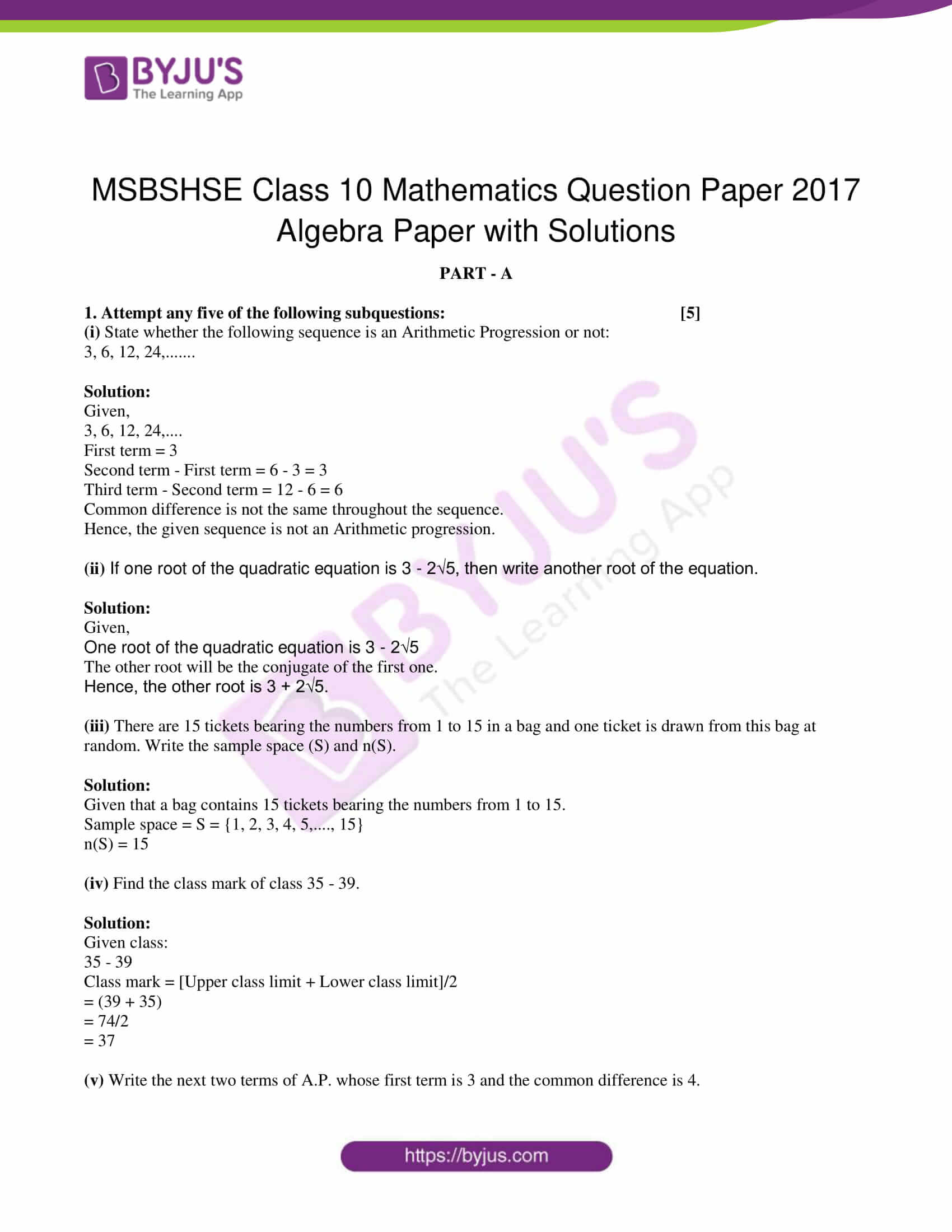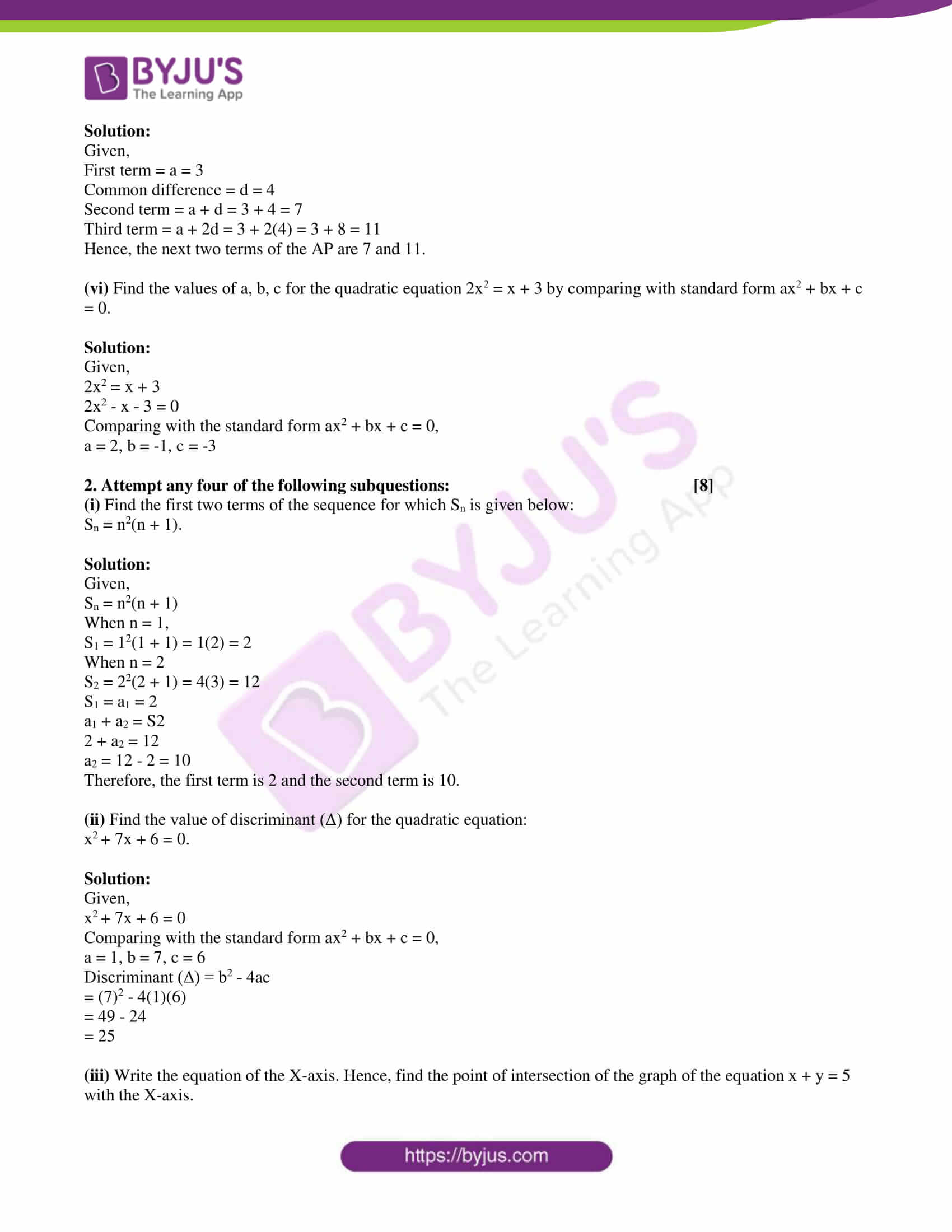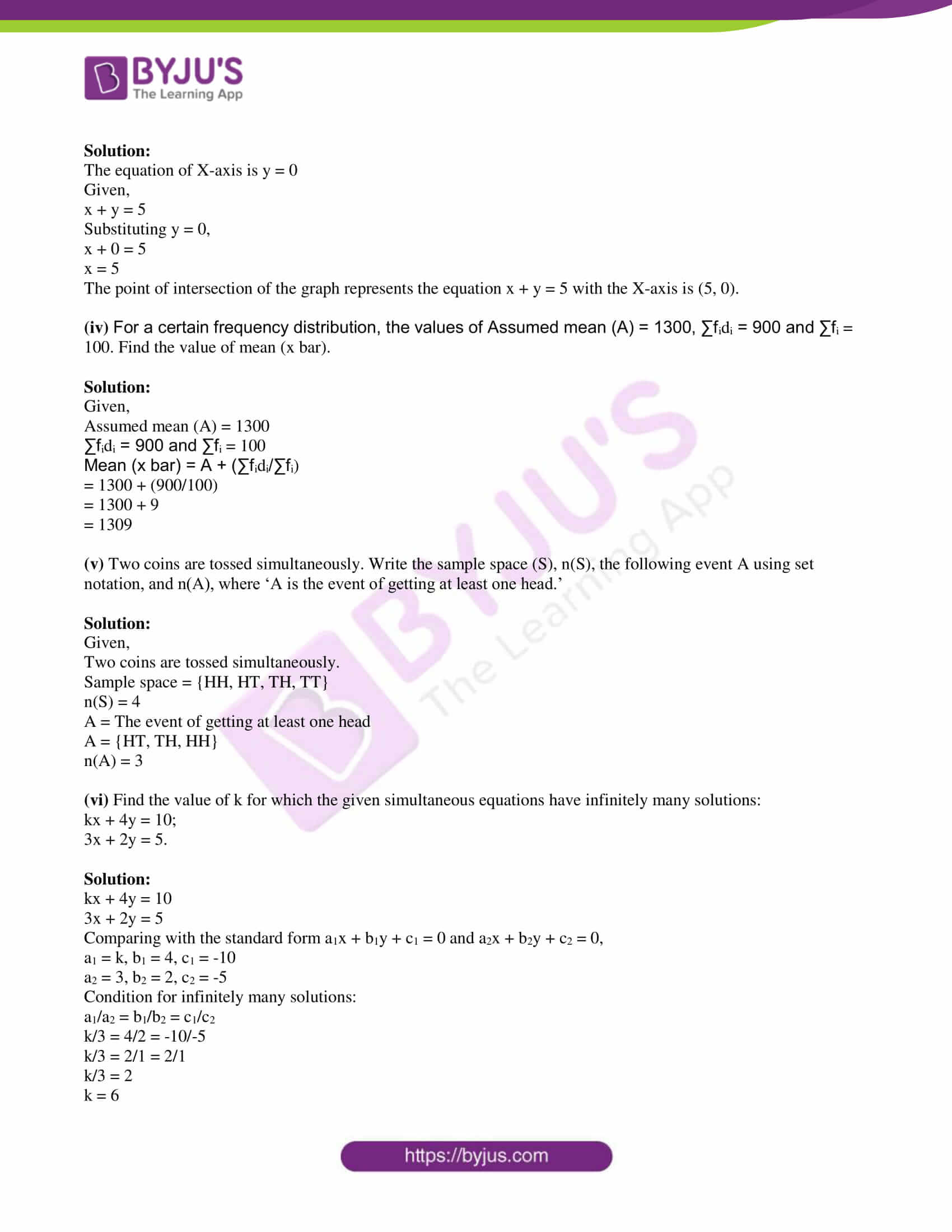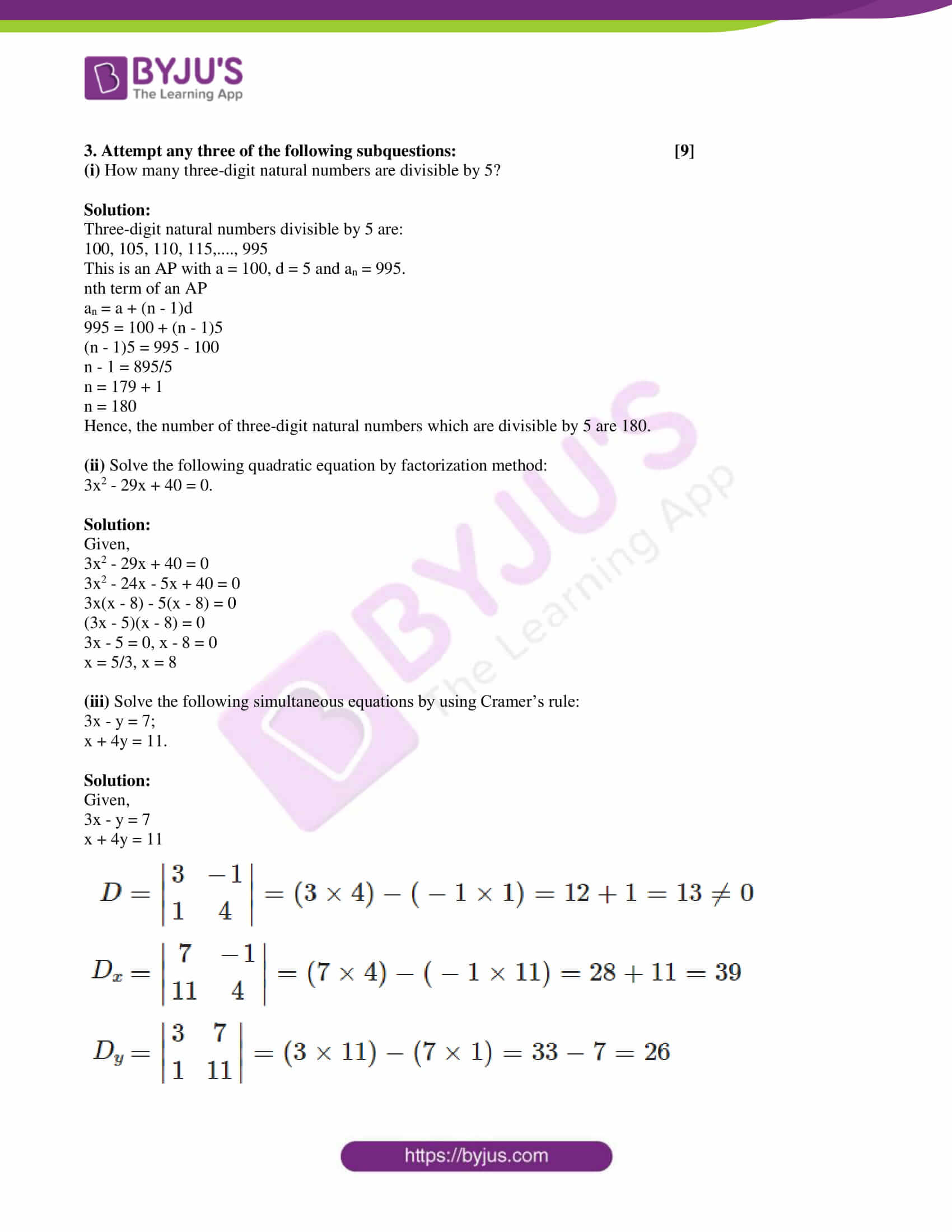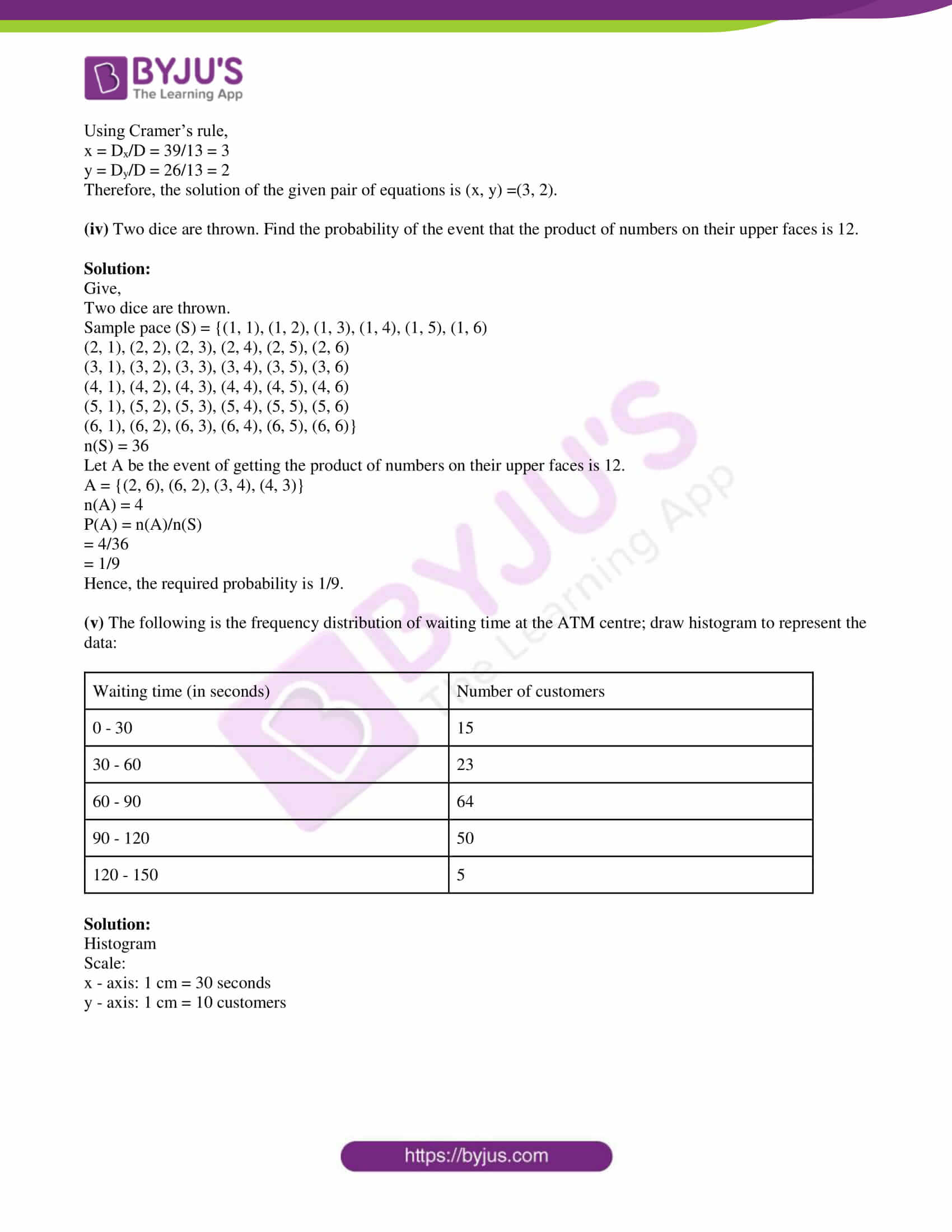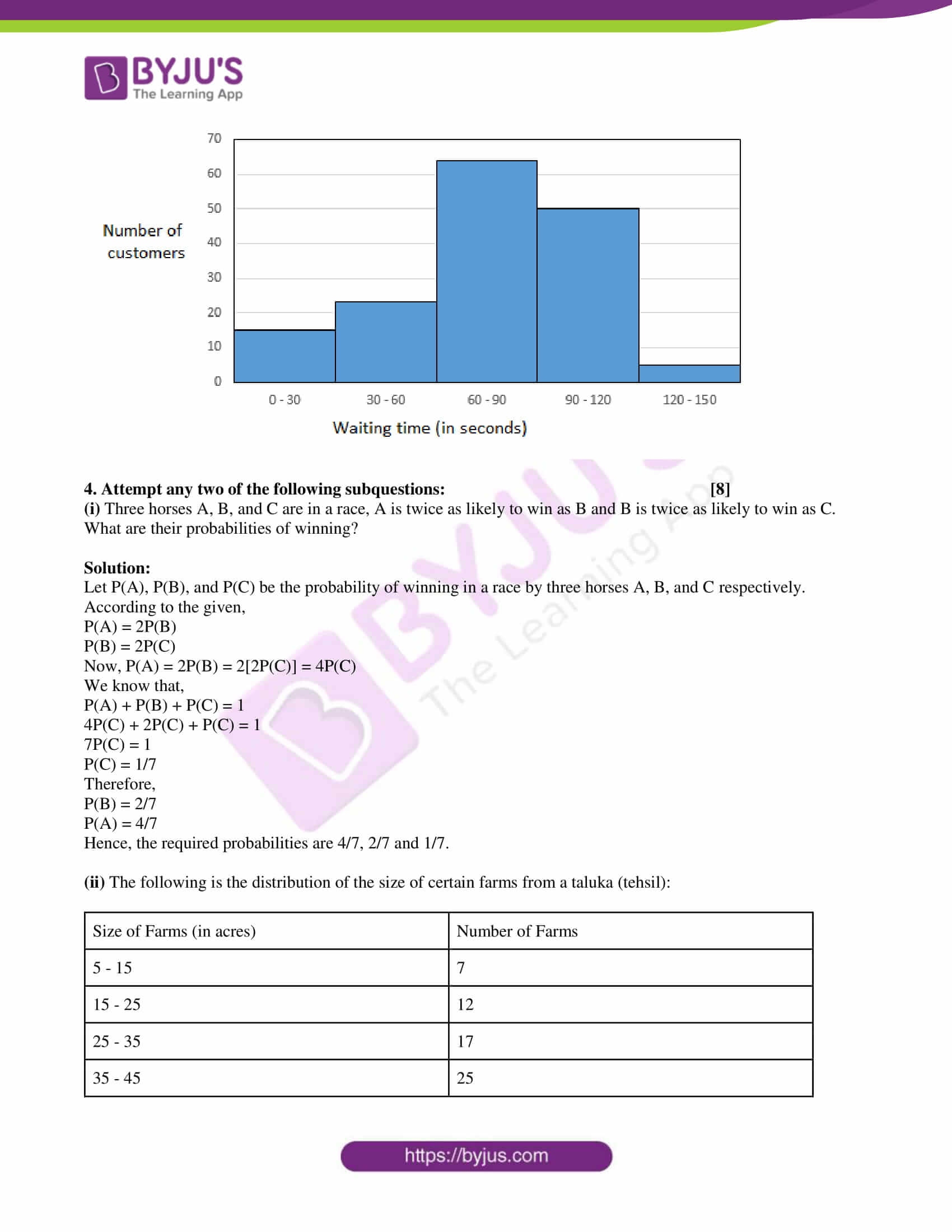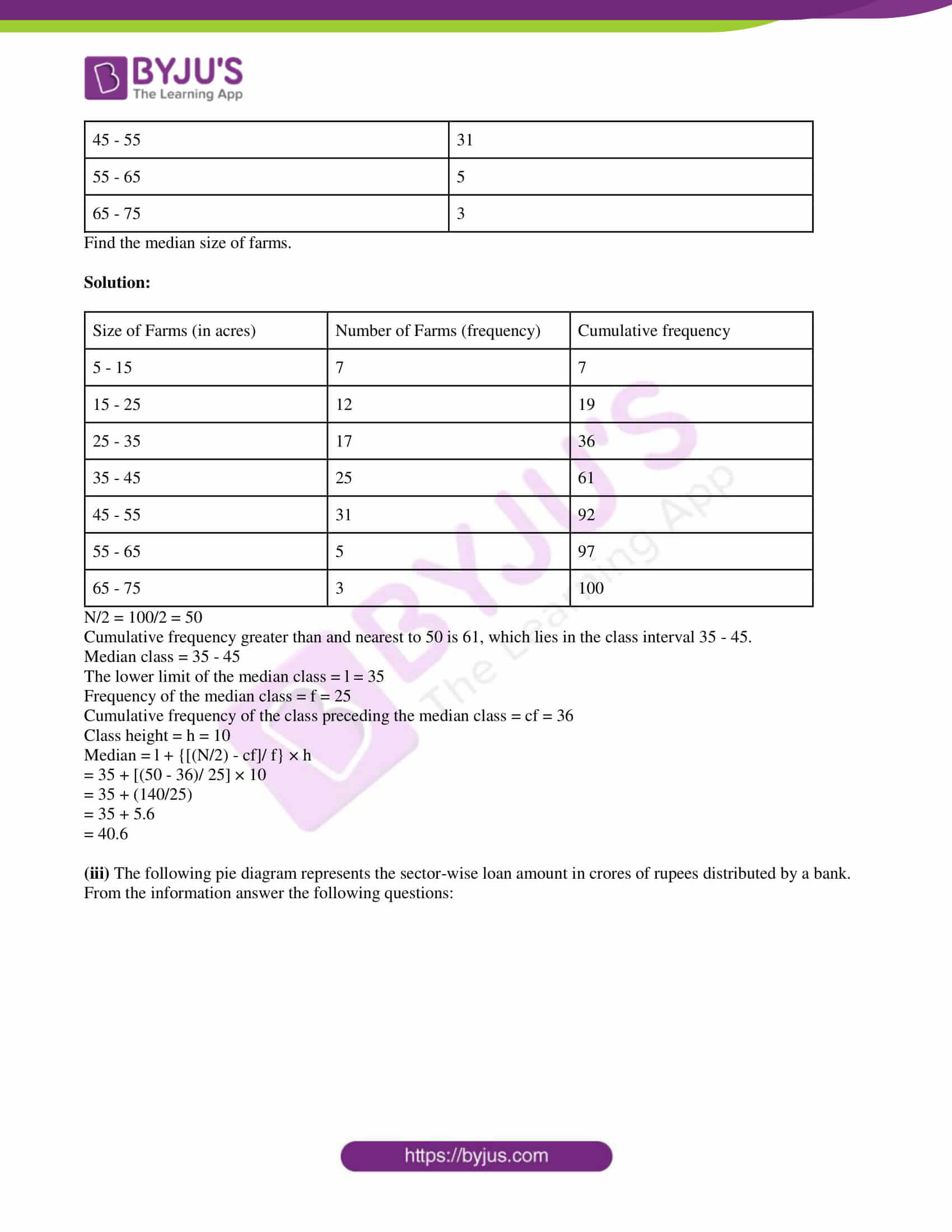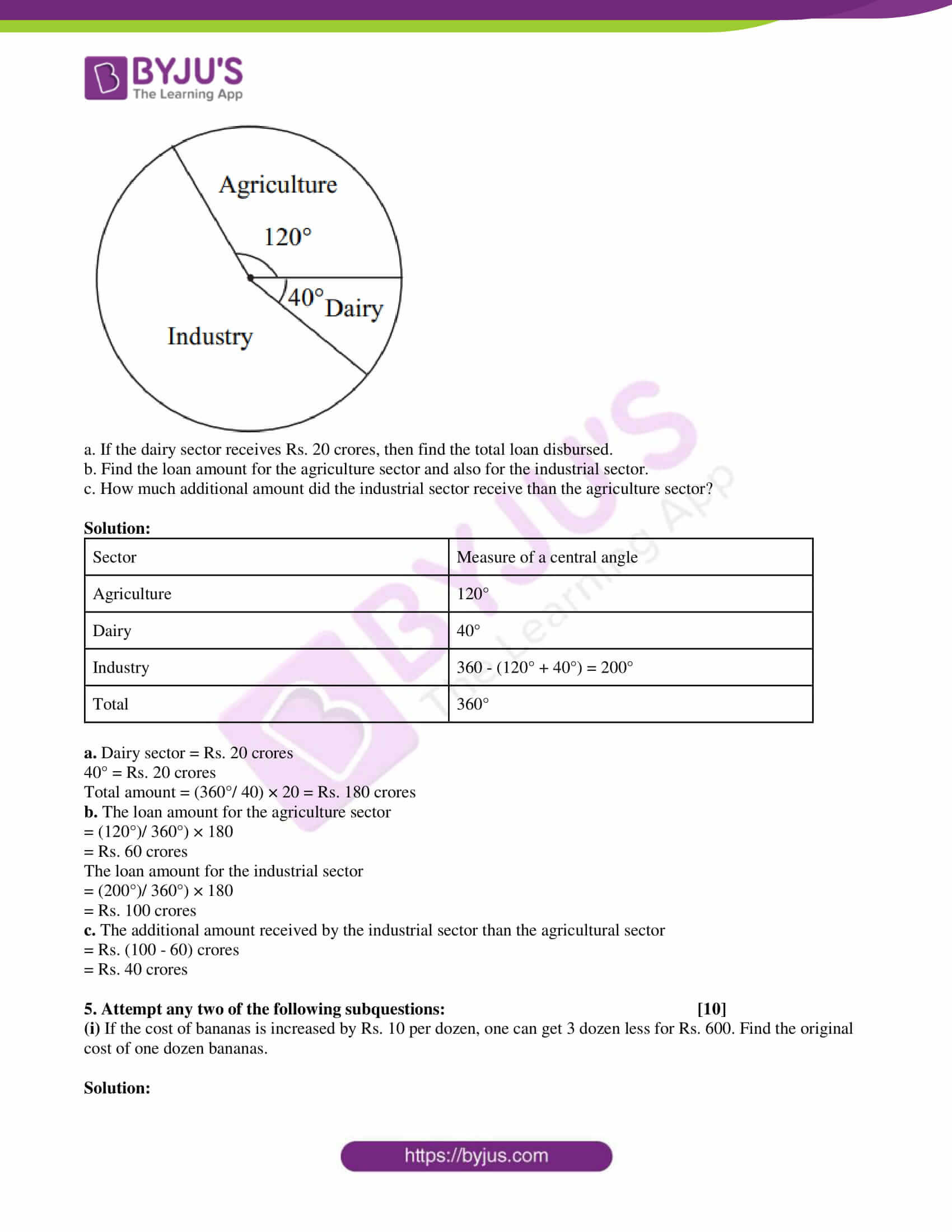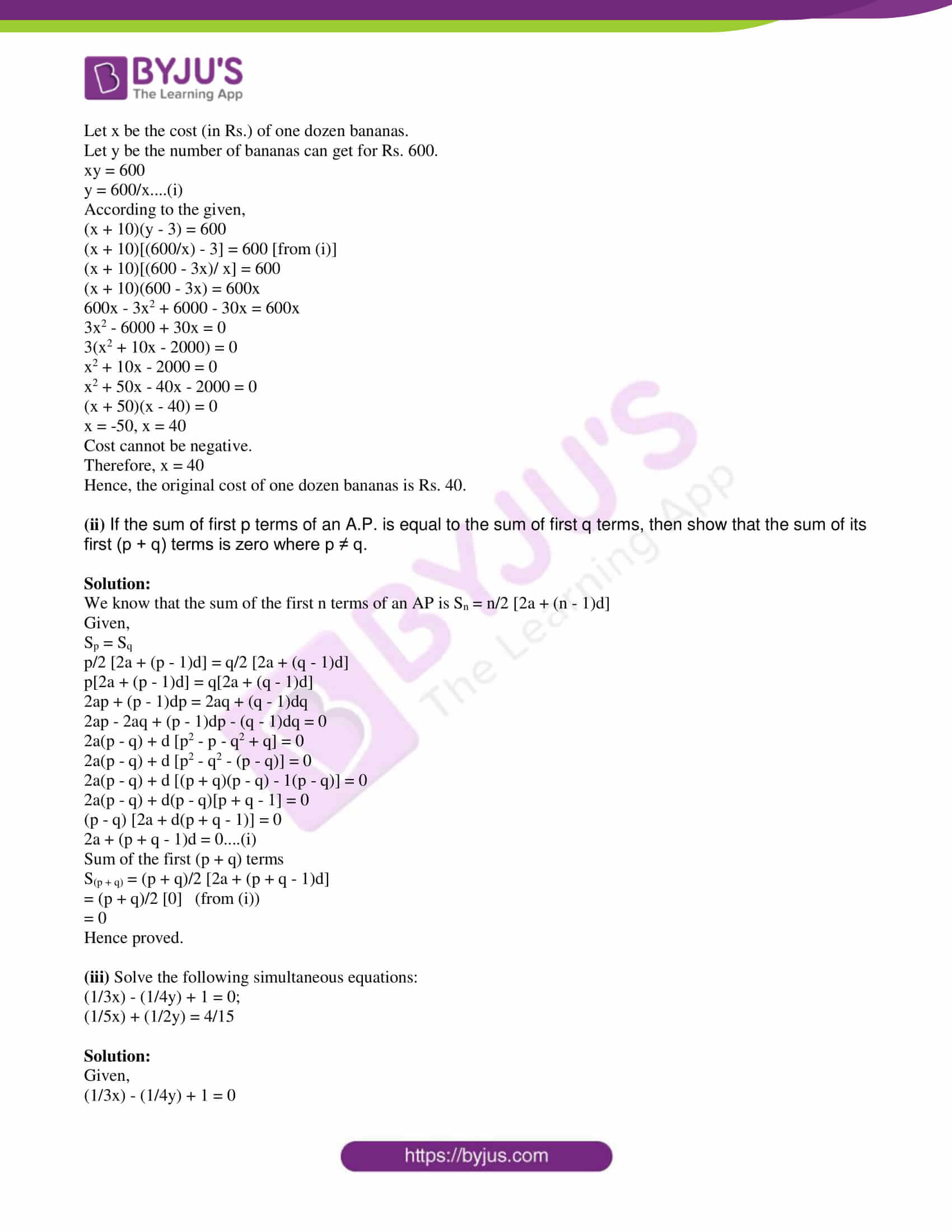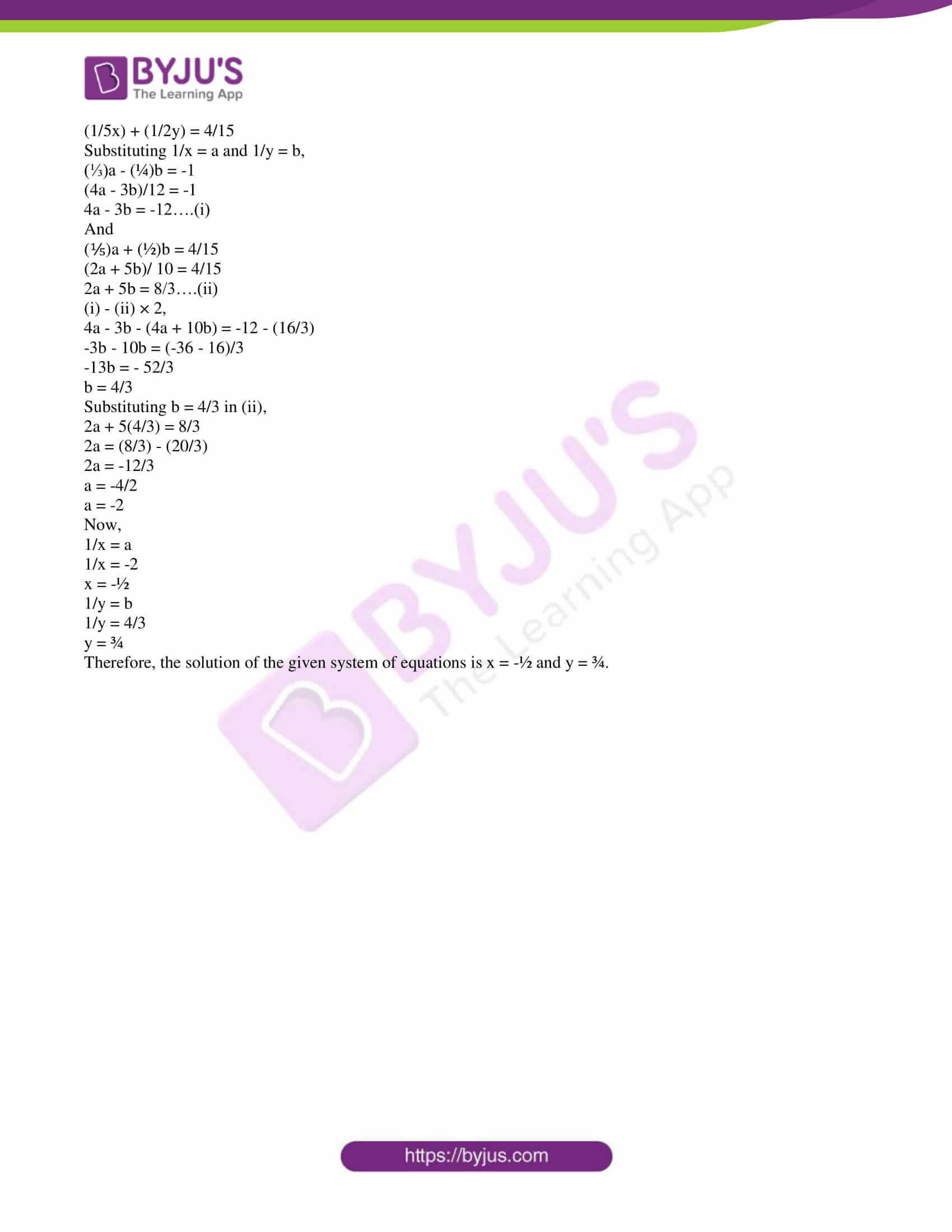PART – A

1. Attempt any five of the following subquestions: 

(i) State whether the following sequence is an Arithmetic Progression or not:

3, 6, 12, 24,…….

Solution:

Given,

3, 6, 12, 24,….

First term = 3

Second term – First term = 6 – 3 = 3

Third term – Second term = 12 – 6 = 6

Common difference is not the same throughout the sequence.

Hence, the given sequence is not an Arithmetic progression.

(ii) If one root of the quadratic equation is 3 – 2√5, then write another root of the equation.

Solution:

Given,

One root of the quadratic equation is 3 – 2√5

The other root will be the conjugate of the first one.

Hence, the other root is 3 + 2√5.

(iii) There are 15 tickets bearing the numbers from 1 to 15 in a bag and one ticket is drawn from this bag at random. Write the sample space (S) and n(S).

Solution:

Given that a bag contains 15 tickets bearing the numbers from 1 to 15.

Sample space = S = {1, 2, 3, 4, 5,…., 15}

n(S) = 15

(iv) Find the class mark of class 35 – 39.

Solution:

Given class:

35 – 39

Class mark = [Upper class limit + Lower class limit]/2

= (39 + 35)/2

= 74/2

= 37

(v) Write the next two terms of A.P. whose first term is 3 and the common difference is 4.

Solution:

Given,

First term = a = 3

Common difference = d = 4

Second term = a + d = 3 + 4 = 7

Third term = a + 2d = 3 + 2(4) = 3 + 8 = 11

Hence, the next two terms of the AP are 7 and 11.

(vi) Find the values of a, b, c for the quadratic equation 2x2 = x + 3 by comparing with standard form ax2 + bx + c = 0.

Solution:

Given,

2x2 = x + 3

2x2 – x – 3 = 0

Comparing with the standard form ax2 + bx + c = 0,

a = 2, b = -1, c = -3

2. Attempt any four of the following subquestions: 

(i) Find the first two terms of the sequence for which Sn is given below:

Sn = n2(n + 1).

Solution:

Given,

Sn = n2(n + 1)

When n = 1,

S1 = 12(1 + 1) = 1(2) = 2

When n = 2

S2 = 22(2 + 1) = 4(3) = 12

S1 = a1 = 2

a1 + a2 = S2

2 + a2 = 12

a2 = 12 – 2 = 10

Therefore, the first term is 2 and the second term is 10.

(ii) Find the value of discriminant (Δ) for the quadratic equation:

x2 + 7x + 6 = 0.

Solution:

Given,

x2 + 7x + 6 = 0

Comparing with the standard form ax2 + bx + c = 0,

a = 1, b = 7, c = 6

Discriminant (Δ) = b2 – 4ac

= (7)2 – 4(1)(6)

= 49 – 24

= 25

(iii) Write the equation of the X-axis. Hence, find the point of intersection of the graph of the equation x + y = 5 with the X-axis.

Solution:

The equation of X-axis is y = 0

Given,

x + y = 5

Substituting y = 0,

x + 0 = 5

x = 5

The point of intersection of the graph represents the equation x + y = 5 with the X-axis is (5, 0).

(iv) For a certain frequency distribution, the values of Assumed mean (A) = 1300, ∑fidi = 900 and ∑fi = 100. Find the value of mean (x bar).

Solution:

Given,

Assumed mean (A) = 1300

∑fidi = 900 and ∑fi = 100

Mean (x bar) = A + (∑fidi/∑fi)

= 1300 + (900/100)

= 1300 + 9

= 1309

(v) Two coins are tossed simultaneously. Write the sample space (S), n(S), the following event A using set notation, and n(A), where ‘A is the event of getting at least one head.’

Solution:

Given,

Two coins are tossed simultaneously.

Sample space = {HH, HT, TH, TT}

n(S) = 4

A = The event of getting at least one head

A = {HT, TH, HH}

n(A) = 3

(vi) Find the value of k for which the given simultaneous equations have infinitely many solutions:

kx + 4y = 10;

3x + 2y = 5.

Solution:

kx + 4y = 10

3x + 2y = 5

Comparing with the standard form a1x + b1y + c1 = 0 and a2x + b2y + c2 = 0,

a1 = k, b1 = 4, c1 = -10

a2 = 3, b2 = 2, c2 = -5

Condition for infinitely many solutions:

a1/a2 = b1/b2 = c1/c2

k/3 = 4/2 = -10/-5

k/3 = 2/1 = 2/1

k/3 = 2

k = 6

3. Attempt any three of the following subquestions: 

(i) How many three-digit natural numbers are divisible by 5?

Solution:

Three-digit natural numbers divisible by 5 are:

100, 105, 110, 115,…., 995

This is an AP with a = 100, d = 5 and an = 995.

nth term of an AP

an = a + (n – 1)d

995 = 100 + (n – 1)5

(n – 1)5 = 995 – 100

n – 1 = 895/5

n = 179 + 1

n = 180

Hence, the number of three-digit natural numbers which are divisible by 5 are 180.

(ii) Solve the following quadratic equation by factorization method:

3x2 – 29x + 40 = 0.

Solution:

Given,

3x2 – 29x + 40 = 0

3x2 – 24x – 5x + 40 = 0

3x(x – 8) – 5(x – 8) = 0

(3x – 5)(x – 8) = 0

3x – 5 = 0, x – 8 = 0

x = 5/3, x = 8

(iii) Solve the following simultaneous equations by using Cramer’s rule:

3x – y = 7;

x + 4y = 11.

Solution:

Given,

3x – y = 7

x + 4y = 11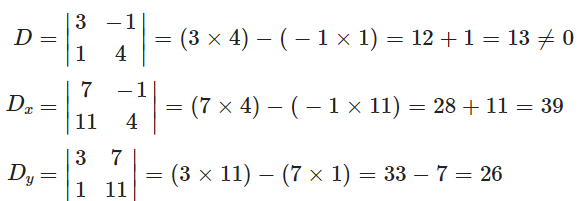Using Cramer’s rule,

x = Dx/D = 39/13 = 3

y = Dy/D = 26/13 = 2

Therefore, the solution of the given pair of equations is (x, y) =(3, 2).

(iv) Two dice are thrown. Find the probability of the event that the product of numbers on their upper faces is 12.

Solution:

Give,

Two dice are thrown.

Sample pace (S) = {(1, 1), (1, 2), (1, 3), (1, 4), (1, 5), (1, 6)

(2, 1), (2, 2), (2, 3), (2, 4), (2, 5), (2, 6)

(3, 1), (3, 2), (3, 3), (3, 4), (3, 5), (3, 6)

(4, 1), (4, 2), (4, 3), (4, 4), (4, 5), (4, 6)

(5, 1), (5, 2), (5, 3), (5, 4), (5, 5), (5, 6)

(6, 1), (6, 2), (6, 3), (6, 4), (6, 5), (6, 6)}

n(S) = 36

Let A be the event of getting the product of numbers on their upper faces is 12.

A = {(2, 6), (6, 2), (3, 4), (4, 3)}

n(A) = 4

P(A) = n(A)/n(S)

= 4/36

= 1/9

Hence, the required probability is 1/9.

(v) The following is the frequency distribution of waiting time at the ATM centre; draw histogram to represent the data:

 Waiting time (in seconds) Number of customers 0 – 30 15 30 – 60 23 60 – 90 64 90 – 120 50 120 – 150 5

Solution:

Histogram

Scale:

x – axis: 1 cm = 30 seconds

y – axis: 1 cm = 10 customers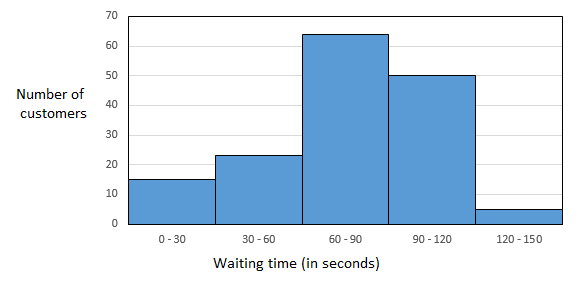4. Attempt any two of the following subquestions: 

(i) Three horses A, B, and C are in a race, A is twice as likely to win as B and B is twice as likely to win as C. What are their probabilities of winning?

Solution:

Let P(A), P(B), and P(C) be the probability of winning in a race by three horses A, B, and C respectively.

According to the given,

P(A) = 2P(B)

P(B) = 2P(C)

Now, P(A) = 2P(B) = 2[2P(C)] = 4P(C)

We know that,

P(A) + P(B) + P(C) = 1

4P(C) + 2P(C) + P(C) = 1

7P(C) = 1

P(C) = 1/7

Therefore,

P(B) = 2/7

P(A) = 4/7

Hence, the required probabilities are 4/7, 2/7 and 1/7.

(ii) The following is the distribution of the size of certain farms from a taluka (tehsil):

 Size of Farms (in acres) Number of Farms 5 – 15 7 15 – 25 12 25 – 35 17 35 – 45 25 45 – 55 31 55 – 65 5 65 – 75 3

Find the median size of farms.

Solution:

 Size of Farms (in acres) Number of Farms (frequency) Cumulative frequency 5 – 15 7 7 15 – 25 12 19 25 – 35 17 36 35 – 45 25 61 45 – 55 31 92 55 – 65 5 97 65 – 75 3 100

N/2 = 100/2 = 50

Cumulative frequency greater than and nearest to 50 is 61, which lies in the class interval 35 – 45.

Median class = 35 – 45

The lower limit of the median class = l = 35

Frequency of the median class = f = 25

Cumulative frequency of the class preceding the median class = cf = 36

Class height = h = 10

Median = l + {[(N/2) – cf]/ f} × h

= 35 + [(50 – 36)/ 25] × 10

= 35 + (140/25)

= 35 + 5.6

= 40.6

(iii) The following pie diagram represents the sector-wise loan amount in crores of rupees distributed by a bank. From the information answer the following questions: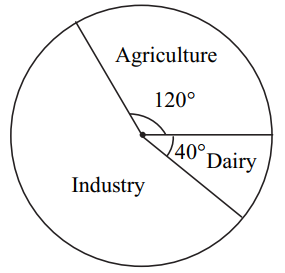a. If the dairy sector receives Rs. 20 crores, then find the total loan disbursed.

b. Find the loan amount for the agriculture sector and also for the industrial sector.

c. How much additional amount did the industrial sector receive than the agriculture sector?

Solution:

 Sector Measure of a central angle Agriculture 120° Dairy 40° Industry 360 – (120° + 40°) = 200° Total 360°

a. Dairy sector = Rs. 20 crores

40° = Rs. 20 crores

Total amount = (360°/ 40) × 20 = Rs. 180 crores

b. The loan amount for the agriculture sector

= (120°)/ 360°) × 180

= Rs. 60 crores

The loan amount for the industrial sector

= (200°)/ 360°) × 180

= Rs. 100 crores

c. The additional amount received by the industrial sector than the agricultural sector

= Rs. (100 – 60) crores

= Rs. 40 crores

5. Attempt any two of the following subquestions: 

(i) If the cost of bananas is increased by Rs. 10 per dozen, one can get 3 dozen less for Rs. 600. Find the original cost of one dozen bananas.

Solution:

Let x be the cost (in Rs.) of one dozen bananas.

Let y be the number of bananas can get for Rs. 600.

xy = 600

y = 600/x….(i)

According to the given,

(x + 10)(y – 3) = 600

(x + 10)[(600/x) – 3] = 600 [from (i)]

(x + 10)[(600 – 3x)/ x] = 600

(x + 10)(600 – 3x) = 600x

600x – 3x2 + 6000 – 30x = 600x

3x2 – 6000 + 30x = 0

3(x2 + 10x – 2000) = 0

x2 + 10x – 2000 = 0

x2 + 50x – 40x – 2000 = 0

(x + 50)(x – 40) = 0

x = -50, x = 40

Cost cannot be negative.

Therefore, x = 40

Hence, the original cost of one dozen bananas is Rs. 40.

(ii) If the sum of first p terms of an A.P. is equal to the sum of first q terms, then show that the sum of its first (p + q) terms is zero where p ≠ q.

Solution:

We know that the sum of the first n terms of an AP is Sn = n/2 [2a + (n – 1)d]

Given,

Sp = Sq

p/2 [2a + (p – 1)d] = q/2 [2a + (q – 1)d]

p[2a + (p – 1)d] = q[2a + (q – 1)d]

2ap + (p – 1)dp = 2aq + (q – 1)dq

2ap – 2aq + (p – 1)dp – (q – 1)dq = 0

2a(p – q) + d [p2 – p – q2 + q] = 0

2a(p – q) + d [p2 – q2 – (p – q)] = 0

2a(p – q) + d [(p + q)(p – q) – 1(p – q)] = 0

2a(p – q) + d(p – q)[p + q – 1] = 0

(p – q) [2a + d(p + q – 1)] = 0

2a + (p + q – 1)d = 0….(i)

Sum of the first (p + q) terms

S(p + q) = (p + q)/2 [2a + (p + q – 1)d]

= (p + q)/2  (from (i))

= 0

Hence proved.

(iii) Solve the following simultaneous equations:

(1/3x) – (1/4y) + 1 = 0;

(1/5x) + (1/2y) = 4/15

Solution:

Given,

(1/3x) – (1/4y) + 1 = 0

(1/5x) + (1/2y) = 4/15

Substituting 1/x = a and 1/y = b,

(⅓)a – (¼)b = -1

(4a – 3b)/12 = -1

4a – 3b = -12….(i)

And

(⅕)a + (½)b = 4/15

(2a + 5b)/ 10 = 4/15

2a + 5b = 8/3….(ii)

(i) – (ii) × 2,

4a – 3b – (4a + 10b) = -12 – (16/3)

-3b – 10b = (-36 – 16)/3

-13b = – 52/3

b = 4/3

Substituting b = 4/3 in (ii),

2a + 5(4/3) = 8/3

2a = (8/3) – (20/3)

2a = -12/3

a = -4/2

a = -2

Now,

1/x = a

1/x = -2

x = -½

1/y = b

1/y = 4/3

y = ¾

Therefore, the solution of the given system of equations is x = -½ and y = ¾.